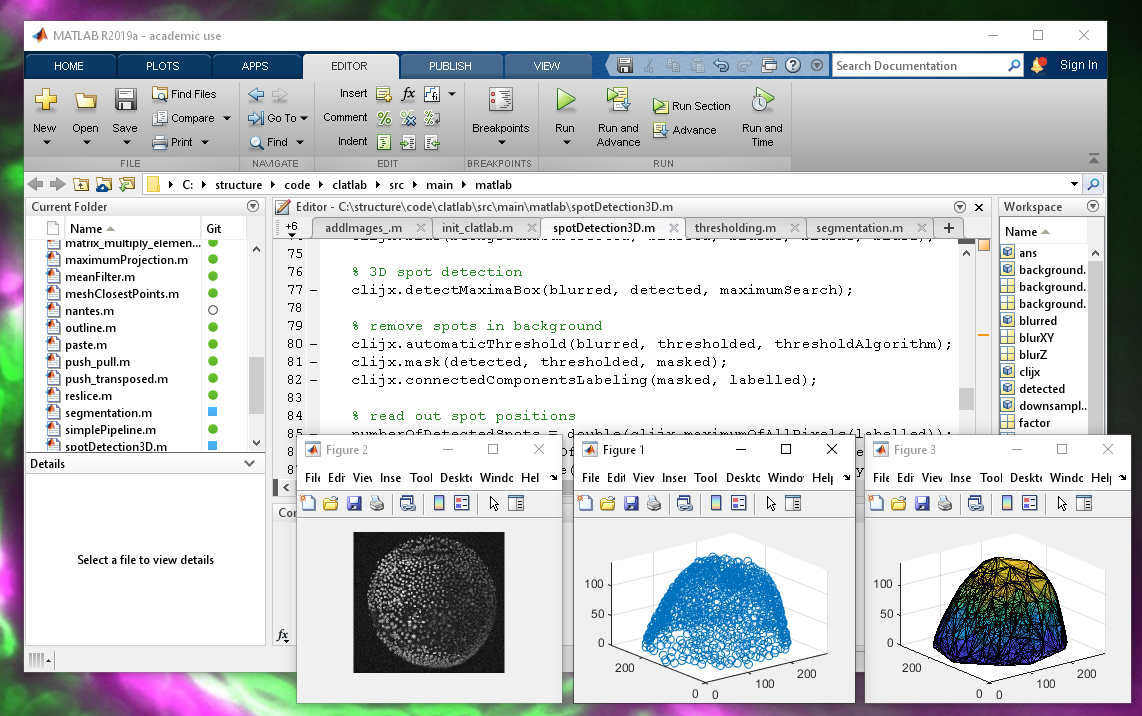# CLATLABGPU-accelerated image processing in Matlab using CLIJ

View the Project on GitHub clij/clatlab

# clatlab

clatlab is a bridge between Matlab and clij2.## Installation

Download the following files, removed the numbers from their file endings and put them all in the `/Documents/MATLAB folder:

Test the installation by executing this script from matlab:

``````clij2 = init_clatlab();
% print out name of used GPU:
clij2.getGPUName()
``````

## Example code

Examples are available in the matlab folder.

Clatlab provides two entry points for processing:

• `clij2` is the entry point to clijs image processing operations. Read the clij2 reference to see which operations are available. Replace `clij.op().` with `clijx` in order to make it run in matlab. For example a Gaussian blur can be applied like this:
``````clij2.gaussianBlur(imageIn, imageOut, 5, 5);
``````
Command Matlab expresson clij2 counter part
push(a)   c = clij2.pushMat(a);
pull(a)   c = clij2.pullMat(a);
plus(a,b) c = a + b clij2.addImages(a, b, c);
minus(a,b) c = a - b clij2.subtractImages(a, b, c);
uminus(a) c = -a clij2.invert(a, c);
transpose(a) c = a.’ clij2.transposeXY(a, c);
times(a,b) c = a .* b clij2.multiplyImages(a, b, c);
mtimes(a,b) c = a * b clij2.multiplyMatrix(a, b, c);
rdivide(a,b) c = a ./ b clij2.divideImages(a, b, c);
ldivide(a,b) c = b ./ b clij2.divideImages(b, a, c);
power(a,b) c = a .^ b clij2.powerImages(a, b, c);
lt(a,b) c = a < b clij2.smaller(a, b, c);
gt(a,b) c = a > b clij2.greater(a, b, c);
le(a,b) c = a <= b clij2.smallerOrEqual(a, b, c);
ge(a,b) c = a >= b clij2.greaterOrEqual(a, b, c);
ne(a,b) c = a ~= b clij2.notEqual(a, b, c);
eq(a,b) c = a == b clij2.equal(a, b, c);
and(a,b) c = a & b clij2.binaryAnd(a, b, c);
or(a,b) c = a I b clij2.binaryOr(a, b, c);
not(a) c = ~b clij2.binaryNot(a, c);
imhist(a) [c,x] = imhist(a) clij2.fillHistogram(a, c);
fliplr(a) c = fliplr(a) clij2.flip(a, c, true, false, false);
min(a) c = min(a) c = clij2.minimumOfAllPixels(a);
max(a) c = max(a) c = clij2.maximumOfAllPixels(a);
mean(a) c = mean(a) c = clij2.meanOfAllPixels(a);
ones(a) c = ones(a) clij2.create(a); clij2.set(a, 1);
zeros(a) c = zeros(a) clij2.create(a); clij2.set(a, 0);
size(a) c = size(a) c = clij2.getDimensions()
colon(a,b) c = [a:b] c = clij2.create(b-a); clij2.setRampX(c);

## How to develop clatlab

Clone this repository and build it using maven. Afterwards, you find the `clatlab.jar` in the `target` directory. Copy it to your clatlab/matlab java classpath.

``````git clone https://github.com/clij/clatlab
cd clatlab
mvn package
cp target/clatlab*.jar classpath/
``````

Back to CLIJ documentation

Imprint